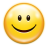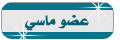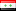منتدى مصر التقني
هل تريد التفاعل مع هذه المساهمة؟ كل ما عليك هو إنشاء حساب جديد ببضع خطوات أو تسجيل الدخول للمتابعة.

# كود لعبة داما في j2me##كود لعبة داما في j2me

اهلا وسهلا بكم في منتدى مصر التقني
-------------------------------------

لعبة داما رائعة للاذكياءكود التالي

الكود:
`import java.util.Vector;import java.io.*;import javax.microedition.io.*;import javax.microedition.rms.*;import javax.microedition.midlet.*;import javax.microedition.lcdui.*;public class Checkers extends MIDlet implements CommandListener {  private CheckersCanvas myCanvas;  private Communicator myCommunicator;  private Command myExitCommand = new Command("Exit", Command.EXIT, 99);  public Checkers() {    try {       myCanvas = new CheckersCanvas(Display.getDisplay(this));      myCanvas.addCommand(myExitCommand);      myCanvas.setCommandListener(this);      CheckersGame game = myCanvas.getGame();     myCommunicator = new Communicator(this, myCanvas, game);      game.setCommunicator(myCommunicator);    } catch(Exception e) {      errorMsg(e);    }  }  public void startApp() throws MIDletStateChangeException {    if(myCanvas != null) {      myCanvas.start();   }    if(myCommunicator != null) {     myCommunicator.start();    } }  public void destroyApp(boolean unconditional)       throws MIDletStateChangeException {    if(myCommunicator != null) {      myCommunicator.endGame();    }    myCommunicator = null;   myCanvas = null;    System.gc();  }  public void pauseApp() {    try {      destroyApp(false);      notifyDestroyed();    } catch (MIDletStateChangeException ex) {    }  }  public void commandAction(Command c, Displayable s) {    if(c == myExitCommand) {      try {          destroyApp(false);          notifyDestroyed();      } catch (MIDletStateChangeException ex) {   }   } }  void errorMsg(Exception e) {    e.printStackTrace();    if(e.getMessage() == null) {      errorMsg(e.getClass().getName());    } else {      errorMsg(e.getMessage());   }  }  void errorMsg(String msg) {    Alert errorAlert = new Alert("error",          msg, null, AlertType.ERROR);    errorAlert.setCommandListener(this);    errorAlert.setTimeout(Alert.FOREVER);    Display.getDisplay(this).setCurrent(errorAlert);  }}class CheckersCanvas extends Canvas {  public static final int BLACK = 0; public static final int WHITE = 0xffffff;  public static final int RED = 0xf96868;  public static final int GREY = 0xc6c6c6;  public static final int LT_GREY = 0xe5e3e3;  public static final int GRID_WIDTH = 8;  private Image myBlackCrown;  private Image myWhiteCrown;  private Display myDisplay;  private CheckersGame myGame;  private int mySquareSize;  private int myMinSquareSize = 15; private boolean myIsWaiting;  CheckersGame getGame() {    return(myGame);  }  void setWaitScreen(boolean wait) {   myIsWaiting = wait;  }  CheckersCanvas(Display d) throws Exception {    myDisplay = d;   myGame = new CheckersGame();   int width = getWidth();  int height = getHeight();    int screenSquareWidth = height;  if(width < height) {      screenSquareWidth = width;    }    mySquareSize = screenSquareWidth ;    if(mySquareSize < myMinSquareSize) {     throw(new Exception("Display too small"));   }    myBlackCrown = Image.createImage("/blackCrown.png");    myWhiteCrown = Image.createImage("/whiteCrown.png");  }  void start() {    myDisplay.setCurrent(this);   myGame.start();  }  protected void paint(Graphics g) {   int width = getWidth();    int height = getHeight();    g.setColor(WHITE);    g.fillRect(0, 0, width, height);    if(myIsWaiting) {     Font font = g.getFont();   int fontHeight = font.getHeight();      int fontWidth = font.stringWidth("waiting for another player");      g.setColor(WHITE);     g.fillRect((width - fontWidth)/2, (height - fontHeight)/2,           fontWidth + 2, fontHeight);      g.setColor(BLACK);     g.setFont(font);     g.drawString("waiting for another player", (width - fontWidth)/2,        (height - fontHeight)/2,       g.TOP|g.LEFT);     return;   }    byte offset = 0;    for(byte i = 0; i < 4; i++) {      for(byte j = 0; j < 8; j++) { if(j % 2 != 0) {    offset = 1;  } else {    offset = 0;  }  if(myGame.isSelected(i, j)) {    g.setColor(LT_GREY);    g.fillRect((2*i + offset)*mySquareSize, j*mySquareSize,      mySquareSize, mySquareSize);  } else {       g.setColor(GREY);    g.fillRect((2*i + offset)*mySquareSize, j*mySquareSize,          mySquareSize, mySquareSize); }  g.setColor(RED);  int piece = myGame.getPiece(i, j);  int circleOffset = 2;  int circleSize = mySquareSize - 2*circleOffset;  if(piece < 0) {    g.setColor(BLACK);    g.fillRoundRect((2*i + offset)*mySquareSize + circleOffset,         j*mySquareSize + circleOffset,        circleSize, circleSize, circleSize, circleSize);    // if the player is a king, draw a crown on:    if(piece < -1) {      g.drawImage(myWhiteCrown,           (2*i + offset)*mySquareSize + mySquareSize/2,           j*mySquareSize + 1 + mySquareSize/2,           Graphics.VCENTER|Graphics.HCENTER);    }  } else if(piece > 0) {    // color the piece in red    g.fillRoundRect((2*i + offset)*mySquareSize + circleOffset,         j*mySquareSize + circleOffset,        circleSize, circleSize, circleSize, circleSize);    // if the player is a king, draw a crown on:    if(piece > 1) {      g.drawImage(myBlackCrown,           (2*i + offset)*mySquareSize + mySquareSize/2,           j*mySquareSize + 1 + mySquareSize/2,           Graphics.VCENTER|Graphics.HCENTER);    }  }      }    }    // now the blank squares:    // actually, this part is probably not necessary...    g.setColor(WHITE);    for(int i = 0; i < 4; i++) {      for(int j = 0; j < 8; j++) {  if(j % 2 == 0) {    offset = 1;  } else {    offset = 0;  }  g.fillRect((2*i + offset)*mySquareSize, j*mySquareSize,        mySquareSize, mySquareSize);      }    }    // if the player has reached the end of the game,     // we display the end message.    if(myGame.getGameOver()) {      // perform some calculations to place the text correctly:      Font font = g.getFont();      int fontHeight = font.getHeight();      int fontWidth = font.stringWidth("Game Over");      g.setColor(WHITE);      g.fillRect((width - fontWidth)/2, (height - fontHeight)/2,           fontWidth + 2, fontHeight);      // write in black      g.setColor(BLACK);      g.setFont(font);      g.drawString("Game Over", (width - fontWidth)/2,        (height - fontHeight)/2,       g.TOP|g.LEFT);    }  }  //-------------------------------------------------------  //  handle keystrokes  /**   * Move the player.   */  public void keyPressed(int keyCode) {      if(myGame.isMyTurn()) {      int action = getGameAction(keyCode);         switch (action) {      case LEFT:  myGame.leftPressed();  break;      case RIGHT:  myGame.rightPressed();  break;      case UP:  myGame.upPressed();  break;      case DOWN:  myGame.deselect();  break;      }      repaint();      serviceRepaints();    }  }}/** * This class contacts a remote server in order to  * play a game of checkers against an opponent.. * * @author Carol Hamer */class Communicator extends Thread {  //--------------------------------------------------------  //  static fields  /**   * This is the URL to contact.   * IMPORTANT: before compiling, the following URL   * must be changed to the correct URL of the    * machine running the server code.   */  public static final String SERVER_URL     = "socket://malbec:8007";  /**   * The int to signal that the game is to begin.   */  public static final byte START_GAME_FLAG = -4;  /**   * The byte to signal that the game is to end.   */  public static final byte END_GAME_FLAG = -3;  /**   * The byte to signal the end of a turn.   */  public static final byte END_TURN_FLAG = -2;  //--------------------------------------------------------  //  game instance fields  /**   * The MIDlet subclass, used to set the Display    * in the case where an error message needs to be sent..   */  private Checkers myCheckers;  /**   * The Canvas subclass, used to set the Display    * in the case where an error message needs to be sent..   */  private CheckersCanvas myCanvas;  /**   * The game logic class that we send the opponent's    * moves to..   */  private CheckersGame myGame;  /**   * Whether or not the MIDlet class has requested the    * game to end.   */  private boolean myShouldStop;  //--------------------------------------------------------  //  data exchange instance fields  /**   * The data from the local player that is to    * be sent to the opponent.   */  private byte[] myMove;  /**   * Whether or not the current turn is done and    * should be sent.   */  private boolean myTurnIsDone = true;  //--------------------------------------------------------  //  initialization  /**   * Constructor is used only when the program wants    * to spawn a data-fetching thread, not for merely    * reading local data with static methods.   */  Communicator(Checkers checkers, CheckersCanvas canvas,          CheckersGame game) {    myCheckers = checkers;    myCanvas = canvas;    myGame = game;  }  //--------------------------------------------------------  //  methods called by CheckersGame to send move  //    information to the opponent.  /**   * Stop the game entirely.  Notify the servlet that    * the user is exiting the game.   */  synchronized void endGame() {    myShouldStop = true;    if(myGame != null) {      myGame.setGameOver();    }    notify();  }  /**   * This is called when the player moves a piece.   */  synchronized void move(byte sourceX, byte sourceY, byte destinationX,         byte destinationY) {    myMove = new byte;    myMove = sourceX;    myMove = sourceY;    myMove = destinationX;    myMove = destinationY;    myTurnIsDone = false;    notify();  }  /**   * This is called when the local player's turn is over.   */  synchronized void endTurn() {    myTurnIsDone = true;    notify();  }  //--------------------------------------------------------  //  main communication method  /**   * Makes a connection to the server and sends and receives   * information about moves.   */  public void run() {    DataInputStream dis = null;    DataOutputStream dos = null;    SocketConnection conn = null;    byte[] fourBytes = new byte;    try {      // tell the user that we're waiting for the other player to join:      myCanvas.setWaitScreen(true);      myCanvas.repaint();      myCanvas.serviceRepaints();      // now make the connection:      conn = (SocketConnection)Connector.open(SERVER_URL);      conn.setSocketOption(SocketConnection.KEEPALIVE, 1);      dos = conn.openDataOutputStream();      dis = conn.openDataInputStream();      // we read four bytes to make sure the connection works...      dis.readFully(fourBytes);      if(fourBytes != START_GAME_FLAG) {  throw(new Exception("server-side error"));      }      // On this line it will block waiting for another       // player to join the game or make a move:      dis.readFully(fourBytes);      // if the server sends the start game flag again,       // that means that we start with the local player's turn.      // Otherwise, we read the other player's first move from the       // stream:      if(fourBytes != START_GAME_FLAG) {  // verify that the other player sent a move   // and not just a message ending the game...  if(fourBytes == END_GAME_FLAG) {    throw(new Exception("other player quit"));  }  // we move the opponent on the local screen.  // then we read from the opponent again,   // in case there's a double-jump:  while(fourBytes != END_TURN_FLAG) {    myGame.moveOpponent(fourBytes);    dis.readFully(fourBytes);  }      }      // now signal the local game that the opponent is done      // so the board must be updated and the local player       // prompted to make a move:      myGame.endOpponentTurn();      myCanvas.setWaitScreen(false);      myCanvas.repaint();      myCanvas.serviceRepaints();      // begin main game loop:      while(! myShouldStop) {  // now it's the local player's turn.  // wait for the player to move a piece:  synchronized(this) {    wait();  }  // after every wait, we check if the game   // ended while we were waiting...  if(myShouldStop) {    break;  }  while(! myTurnIsDone) {    // send the current move:    if(myMove != null) {      dos.write(myMove, 0, myMove.length);      myMove = null;    }    // If the player can continue the move with a double     // jump, we wait for the player to do it:    synchronized(this) {      // make sure the turn isn't done before we start waiting      // (the end turn notify might accidentally be called       // before we start waiting...)      if(! myTurnIsDone) {        wait();      }     }  }  // after every wait, we check if the game   // ended while we were waiting...  if(myShouldStop) {    break;  }  // now we tell the other player the this player's   // turn is over:  fourBytes = END_TURN_FLAG;  dos.write(fourBytes, 0, fourBytes.length);  // now that we've sent the move, we wait for a response:  dis.readFully(fourBytes);  while((fourBytes != END_TURN_FLAG) &&         (fourBytes != END_GAME_FLAG) && (!myShouldStop)) {    // we move the opponent on the local screen.    // then we read from the opponent again,     // in case there's a double-jump:    myGame.moveOpponent(fourBytes);    dis.readFully(fourBytes);  }  // if the other player has left the game, we tell the   // local user that the game is over.  if((fourBytes == END_GAME_FLAG) || (myShouldStop)) {    endGame();    break;  }  myGame.endOpponentTurn();  myCanvas.repaint();  myCanvas.serviceRepaints();      } // end while loop    } catch(Exception e) {      // if there's an error, we display its messsage and       // end the game.      myCheckers.errorMsg(e.getMessage());    } finally {      // now we send the information that we're leaving the game,      // then close up and delete everything.      try {  if(dos != null) {    dos.write(END_GAME_FLAG);    dos.close();  }  if(dis != null) {    dis.close();  }  if(conn != null) {    conn.close();  }  dis = null;  dos = null;  conn = null;      } catch(Exception e) {  // if this throws, at least we made our best effort   // to close everything up....      }    }    // one last paint job to display the "Game Over"    myCanvas.repaint();    myCanvas.serviceRepaints();  }    }/** * This class is a set of simple utility functions that  * can be used to convert standard data types to bytes  * and back again.  It is used especially for data storage,  * but also for sending and receiving data. *  * @author Carol Hamer */class DataConverter {  //--------------------------------------------------------  //  utilities to encode small, compactly-stored small ints.  /**   * Encodes a coordinate pair into a byte.   * @param coordPair a pair of integers to be compacted into   * a single byte for storage.   * WARNING: each of the two values MUST BE    * between 0 and 15 (inclusive).  This method does not    * verify the length of the array (which must be 2!)    * nor does it verify that the ints are of the right size.   */  public static byte encodeCoords(int[] coordPair) {    // get the byte value of the first coordinate:    byte retVal = (new Integer(coordPair)).byteValue();    // move the first coordinate's value up to the top     // half of the storage byte:    retVal = (new Integer(retVal << 4)).byteValue();    // store the second coordinate in the lower half    // of the byte:    retVal += (new Integer(coordPair)).byteValue();    return(retVal);  }  /**   * Encodes eight ints into a byte.   * This could be easily modified to encode eight booleans.   * @param eight an array of at least eight ints.   * WARNING: all values must be 0 or 1!  This method does    * not verify that the values are in the correct range    * nor does it verify that the array is long enough.   * @param offset the index in the array eight to start   * reading data from.  (should usually be 0)   */  public static byte encode8(int[] eight, int offset) {    // get the byte value of the first int:    byte retVal = (new Integer(eight[offset])).byteValue();    // progressively move the data up one bit in the     // storage byte and then record the next int in    // the lowest spot in the storage byte:    for(int i = offset + 1; i < 8 + offset; i++) {      retVal = (new Integer(retVal << 1)).byteValue();      retVal += (new Integer(eight[i])).byteValue();    }    return(retVal);  }  //--------------------------------------------------------  //  utilities to decode small, compactly-stored small ints.  /**   * Turns a byte into a pair of coordinates.   */  public static int[] decodeCoords(byte coordByte) {    int[] retArray = new int;    // we perform a bitwise and with the value 15     // in order to just get the bits of the lower    // half of the byte:    retArray = coordByte & 15;    // To get the bits of the upper half of the     // byte, we perform a shift to move them down:    retArray = coordByte >> 4;    // bytes in Java are generally assumed to be     // signed, but in this coding algorithm we     // would like to treat them as unsigned:     if(retArray < 0) {      retArray += 16;    }    return(retArray);  }  /**   * Turns a byte into eight ints.   */  public static int[] decode8(byte data) {    int[] retArray = new int;    // The flag allows us to look at each bit individually    // to determine if it is 1 or 0.  The number 128     // corresponds to the highest bit of a byte, so we     // start with that one.    int flag = 128;    // We use a loop that checks     // the data bit by bit by performing a bitwise     // and (&) between the data byte and a flag:    for(int i = 0; i < 8; i++) {      if((flag & data) != 0) {  retArray[i] = 1;      } else {  retArray[i] = 0;      }      // move the flag down one bit so that we can       // check the next bit of data on the next pass      // through the loop:      flag = flag >> 1;    }    return(retArray);  }  //--------------------------------------------------------  //  standard integer interpretation  /**   * Uses an input stream to convert an array of bytes to an int.   */  public static int parseInt(byte[] data) throws IOException {    DataInputStream stream       = new DataInputStream(new ByteArrayInputStream(data));    int retVal = stream.readInt();    stream.close();    return(retVal);  }  /**   * Uses an output stream to convert an int to four bytes.   */  public static byte[] intToFourBytes(int i) throws IOException {    ByteArrayOutputStream baos = new ByteArrayOutputStream(4);    DataOutputStream dos = new DataOutputStream(baos);    dos.writeInt(i);    baos.close();    dos.close();    byte[] retArray = baos.toByteArray();    return(retArray);  }  //--------------------------------------------------------  //  integer interpretation illustrated  /**   * Java appears to treat a byte as being signed when   * returning it as an int--this function converts from   * the signed value to the corresponding unsigned value.   * This method is used by nostreamParseInt.   */  public static int unsign(int signed) {    int retVal = signed;    if(retVal < 0) {      retVal += 256;    }    return(retVal);  }  /**   * Takes an array of bytes and returns an int.   * This version will return the same value as the    * method parseInt above.  This version is included    * in order to illustrate how Java encodes int values   * in terms of bytes.   * @param data an array of 1, 2, or 4 bytes.   */  public static int nostreamParseInt(byte[] data) {    // byte 0 is the high byte which is assumed     // to be signed.  As we add the lower bytes     // one by one, we unsign them because because     // a single byte alone is interpreted as signed,     // but in an int only the top byte should be signed.    // (note that the high byte is the first one in the array)    int retVal = data;    for(int i = 1; i < data.length; i++) {      retVal = retVal << 8;      retVal += unsign(data[i]);    }    return(retVal);  }  /**   * Takes an arbitrary int and returns   * an array of four bytes.   * This version will return the same byte array    * as the method intToFourBytes above.  This version    * is included in order to illustrate how Java encodes    * int values in terms of bytes.   */  public static byte[] nostreamIntToFourBytes(int i) {    byte[] fourBytes = new byte;    // when you take the byte value of an int, it    // only gives you the lowest byte.  So we     // get all four bytes by taking the lowest     // byte four times and moving the whole int     // down by one byte between each one.    // (note that the high byte is the first one in the array)    fourBytes = (new Integer(i)).byteValue();    i = i >> 8;    fourBytes = (new Integer(i)).byteValue();    i = i >> 8;    fourBytes = (new Integer(i)).byteValue();    i = i >> 8;    fourBytes = (new Integer(i)).byteValue();    return(fourBytes);  }  /**   * Takes an int between -32768 and 32767 and returns   * an array of two bytes.  This does not verify that    * the argument is of the right size.  If the absolute   * value of i is too high, it will not be encoded    * correctly.   */  public static byte[] nostreamIntToTwoBytes(int i) {    byte[] twoBytes = new byte;    // when you take the byte value of an int, it    // only gives you the lowest byte.  So we     // get the lower two bytes by taking the lowest     // byte twice and moving the whole int     // down by one byte between each one.    twoBytes = (new Integer(i)).byteValue();    i = i >> 8;    twoBytes = (new Integer(i)).byteValue();    return(twoBytes);  }}/** * This class takes care of the underlying logic and data of  * the checkers game being played.  That includes where  * all of the pieces are on the board and where it is okay  * for them to move to.   * * @author Carol Hamer */class CheckersGame {  //-------------------------------------------------------  //   static fields  /**   * The length of the checkerboard in the x-direction.   */  public static final byte X_LENGTH = 4;  /**   * The length of the checkerboard in the y-direction.   */  public static final byte Y_LENGTH = 8;  //-------------------------------------------------------  //   instance fields  /**   * a handle to the communications class that exchanges   * data with the server.   */  private Communicator myCommunicator;  /**   * This array represents the black squares of the    * checkerboard.  The two dimensions of the array    * represent the two dimensions of the checkerboard.   * The value represents what type of piece is on    * the square.   * 0 = empty   * 1 = local player's piece   * 2 = local player's king   * -1 = remote player's piece   * -2 = remote player's king   */  private byte[][] myGrid;  /**   * If the user has currently selected a piece to move,    * this is its X grid coordinate. (-1 if none selected)   */  private byte mySelectedX = -1;  /**   * If the user has currently selected a piece to move,    * this is its Y grid coordinate.(-1 if none selected)   */  private byte mySelectedY = -1;  /**   * If the user has currently selected a possible    * destination square for a move, this is its X coordinate..   * (-1 if none selected)   */  private byte myDestinationX = -1;  /**   * If the user has currently selected a possible    * destination square for a move, this is its Y coordinate..   * (-1 if none selected)   */  private byte myDestinationY = -1;  /**   * This Vector contains the coordinates of all of the    * squares that the player could currently move to.   */  private Vector myPossibleMoves = new Vector(4);  /**   * Whether or not the currently displayed checkers has    * been completed.   */  private boolean myGameOver = false;  /**   * Whether or not it is currently this player's turn.   */  private boolean myTurn = false;  /**   * This is true if the player has just jumped and can    * jump again.   */  private boolean myIsJumping = false;  //-------------------------------------------------------  //   get/set data    /**   * get the piece on the given grid square.   */  byte getPiece(byte x, byte y) {    return(myGrid[x][y]);  }  /**   * This is callsed by CheckersCanvas to determine if    * the square is currently selected (as containing    * a piece to move or a destination square).   */  boolean isSelected(byte x, byte y) {    boolean retVal = false;    if((x == mySelectedX) && (y == mySelectedY)) {      retVal = true;    } else if((x == myDestinationX) && (y == myDestinationY)) {      retVal = true;    }    return(retVal);  }  /**   * This tells whether or not the keystrokes should currently   * be taken into account.   */  boolean isMyTurn() {    boolean retVal = false;    if((!myGameOver) && ((myTurn) || (myIsJumping))) {      retVal = true;    }    return(retVal);  }  /**   * This tells whether or not the game has ended.   */  boolean getGameOver() {    boolean retVal = false;    if(myGameOver) {      retVal = true;    }    return(retVal);  }  /**   * tell the CheckersGame that the other player has ended the game.   */  void setGameOver() {    myGameOver = true;  }  /**   * set the communicator object.   */  void setCommunicator(Communicator comm) {    myCommunicator = comm;  }  //-------------------------------------------------------  //   initialization  /**   * Constructor puts the pieces in their initial positions:   */  CheckersGame() {    myGrid = new byte[X_LENGTH][];    for(byte i = 0; i < myGrid.length; i++) {      myGrid[i] = new byte[Y_LENGTH];      for(byte j = 0; j < myGrid[i].length; j++) {  if(j < 3) {    // fill the top of the board with remote players    myGrid[i][j] = -1;  } else if(j > 4) {    // fill the bottom of the board with local players    myGrid[i][j] = 1;  }      }    }  }  /**   * This is called just before the player makes the    * first move.   */  void start() {    mySelectedX = 0;    mySelectedY = 5;    myTurn = true;    getMoves(mySelectedX, mySelectedY, myPossibleMoves, false);  }  //-------------------------------------------------------  //   move the opponent  // to be called by Communicator  /**   * This is called when the opponent wants to move   * its piece.   * @param moveData an array of four bytes:   * moveData = opponent's initial X coordinate   * moveData = opponent's initial Y coordinate   * moveData = opponent's destination X coordinate   * moveData = opponent's destination Y coordinate   */  void moveOpponent(byte[] moveData) {    // since both players appear on their own screens     // as the red side (bottom of the screen), we need     // to invert the opponent's move:    moveData = (new Integer(X_LENGTH - moveData - 1)).byteValue();    moveData = (new Integer(X_LENGTH - moveData - 1)).byteValue();    moveData = (new Integer(Y_LENGTH - moveData - 1)).byteValue();    moveData = (new Integer(Y_LENGTH - moveData - 1)).byteValue();    myGrid[moveData][moveData]       = myGrid[moveData][moveData];    myGrid[moveData][moveData] = 0;    // deal with an opponent's jump:    if((moveData - moveData > 1) ||        (moveData - moveData > 1)) {      int jumpedY = (moveData + moveData)/2;      int jumpedX = moveData;      int parity = moveData % 2;      if((parity > 0) && (moveData > moveData)) {  jumpedX++;      } else if((parity == 0) && (moveData > moveData)) {  jumpedX--;      }      myGrid[jumpedX][jumpedY] = 0;    }    // if the opponent reaches the far side,     // make him a king:    if(moveData == Y_LENGTH - 1) {      myGrid[moveData][moveData] = -2;    }  }  /**   * This is called when the opponent's turn is over.   * Note that the turn doesn't automatically end after    * the opponent moves because the opponent may make    * a double or triple jump.   */  void endOpponentTurn() {    myTurn = true;    // Now begin the local player's turn:     // First select the first local piece that can be     // moved. (rightPressed will select an appropriate     // piece or end the game if the local player has     // no possible moves to make)    mySelectedX = 0;    mySelectedY = 0;    myDestinationX = -1;    myDestinationY = -1;    rightPressed();    // the local player's thread has been waiting     // for the opponent's turn to end.      synchronized(this) {      notify();    }  }  //-------------------------------------------------------  //   handle keystrokes  // to be called by CheckersCanvas  /**   * if the left button is pressed, this method takes    * the correct course of action depending on the situation.   */  void leftPressed() {    // in the first case the user has not yet selected a     // piece to move:    if(myDestinationX == -1) {      // find the next possible piece (to the left)       // that can move:      selectPrevious();      // if selectPrevious fails to fill myPossibleMoves, that       // means that the local player cannot move, so the game      // is over:      if(myPossibleMoves.size() == 0) {  myCommunicator.endGame();      }    } else {      // if the user has already selected a piece to move,       // we give the options of where the piece can move to:      for(byte i = 0; i < myPossibleMoves.size(); i++) {  byte[] coordinates = (byte[])myPossibleMoves.elementAt(i);  if((coordinates == myDestinationX) &&      (coordinates == myDestinationY)) {    i++;    i = (new Integer(i % myPossibleMoves.size())).byteValue();    coordinates = (byte[])myPossibleMoves.elementAt(i);    myDestinationX = coordinates;    myDestinationY = coordinates;    break;  }      }    }  }  /**   * if the left button is pressed, this method takes    * the correct course of action depending on the situation.   */  void rightPressed() {    // in the first case the user has not yet selected a     // piece to move:    if(myDestinationX == -1) {      // find the next possible piece that can       // move:      selectNext();      // if selectNext fails to fill myPossibleMoves, that       // means that the local player cannot move, so the game      // is over:      if(myPossibleMoves.size() == 0) {  myCommunicator.endGame();      }    } else {      // if the user has already selected a piece to move,       // we give the options of where the piece can move to:      for(byte i = 0; i < myPossibleMoves.size(); i++) {  byte[] coordinates = (byte[])myPossibleMoves.elementAt(i);  if((coordinates == myDestinationX) &&      (coordinates == myDestinationY)) {    i++;    i = (new Integer(i % myPossibleMoves.size())).byteValue();    coordinates = (byte[])myPossibleMoves.elementAt(i);    myDestinationX = coordinates;    myDestinationY = coordinates;    break;  }      }    }  }  /**   * If no piece is selected, we select one.  If a piece    * is selected, we move it.   */  void upPressed() {    // in the first case the user has not yet selected a     // piece to move:    if(myDestinationX == -1) {      fixSelection();    } else {      // if the source square and destination square       // have been chosen, we move the piece:      move();    }  }  /**   * If the user decided not to move the selected piece    * (and instead wants to select again), this undoes    * the selection. This corresponds to pressing the    * DOWN key.   */  void deselect() {    // if the player has just completed a jump and     // could possibly jump again but decides not to     // (i.e. deselects), then the turn ends:    if(myIsJumping) {      mySelectedX = -1;      mySelectedY = -1;      myDestinationX = -1;      myDestinationY = -1;      myIsJumping = false;      myTurn = false;      myCommunicator.endTurn();    } else {      // setting the destination coordinates to -1       // is the signal that the the choice of which       // piece to move can be modified:      myDestinationX = -1;      myDestinationY = -1;    }  }  //-------------------------------------------------------  //   internal square selection methods  /**   * When the player has decided that the currently selected   * square contains the piece he really wants to move, this    * is called. This method switches to the mode where    * the player selects the destination square of the move.   */  private void fixSelection() {    byte[] destination = (byte[])myPossibleMoves.elementAt(0);    // setting the destination coordinates to valid     // coordinates is the signal that the user is done     // selecting the piece to move and now is choosing     // the destination square:    myDestinationX = destination;    myDestinationY = destination;  }  /**   * This method starts from the currently selected square    * and finds the next square that contains a piece that    * the player can move.   */  private void selectNext() {    // Test the squares one by one (starting from the     // currently selected square) until we find a square     // that contains one of the local player's pieces     // that can move:    byte testX = mySelectedX;    byte testY = mySelectedY;    while(true) {      testX++;      if(testX >= X_LENGTH) {  testX = 0;  testY++;  testY = (new Integer(testY % Y_LENGTH)).byteValue();      }      getMoves(testX, testY, myPossibleMoves, false);      if((myPossibleMoves.size() != 0) ||      ((testX == mySelectedX) && (testY == mySelectedY))) {  mySelectedX = testX;  mySelectedY = testY;  break;      }    }  }  /**   * This method starts from the currently selected square    * and finds the next square (to the left) that contains    * a piece that the player can move.   */  private void selectPrevious() {    // Test the squares one by one (starting from the     // currently selected square) until we find a square     // that contains one of the local player's pieces     // that can move:    byte testX = mySelectedX;    byte testY = mySelectedY;    while(true) {      testX--;      if(testX < 0) {  testX += X_LENGTH;  testY--;  if(testY < 0) {    testY += Y_LENGTH;  }      }      getMoves(testX, testY, myPossibleMoves, false);      if((myPossibleMoves.size() != 0) ||    ((testX == mySelectedX) && (testY == mySelectedY))) {  mySelectedX = testX;  mySelectedY = testY;  break;      }    }  }  //-------------------------------------------------------  //   internal utilities  /**   * Once the user has selected the move to make, this    * updates the data accordingly.   */  private void move() {    // the piece that was on the source square is     // now on the destination square:    myGrid[myDestinationX][myDestinationY]       = myGrid[mySelectedX][mySelectedY];    // the source square is emptied:    myGrid[mySelectedX][mySelectedY] = 0;    if(myDestinationY == 0) {      myGrid[myDestinationX][myDestinationY] = 2;    }    // tell the communicator to inform the other player     // of this move:    myCommunicator.move(mySelectedX, mySelectedY,       myDestinationX, myDestinationY);    // deal with the special rules for jumps::    if((mySelectedY - myDestinationY > 1) ||        (myDestinationY - mySelectedY > 1)) {      int jumpedY = (mySelectedY + myDestinationY)/2;      int jumpedX = mySelectedX;      int parity = mySelectedY % 2;      // the coordinates of the jumped square depend on       // what row we're in:      if((parity > 0) && (myDestinationX > mySelectedX)) {          jumpedX++;      } else if((parity == 0) && (mySelectedX > myDestinationX)) {          jumpedX--;      }      // remove the piece that was jumped over:      myGrid[jumpedX][jumpedY] = 0;      mySelectedX = myDestinationX;      mySelectedY = myDestinationY;      myDestinationX = -1;      myDestinationY = -1;`

عدل سابقا من قبل أحمد مناع في الأربعاء فبراير 05, 2014 9:24 am عدل 1 مرات (السبب : للتنسيق)أيمن الإبراهيم
........تاريخ التسجيل : 19/03/2013
المساهمات : 251
النقاط : 398
التقيم : 1
الدولة :الجنس :ََ

# مواضيع ذات صلة

صلاحيات هذا المنتدى:
لاتستطيع الرد على المواضيع في هذا المنتدى